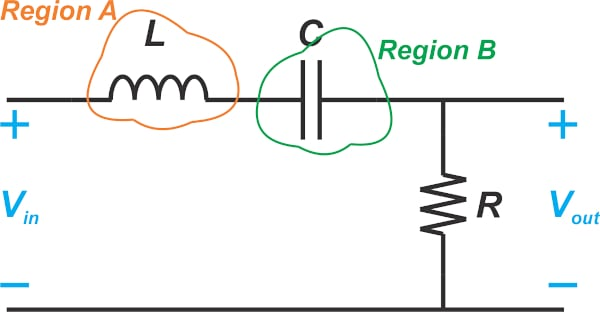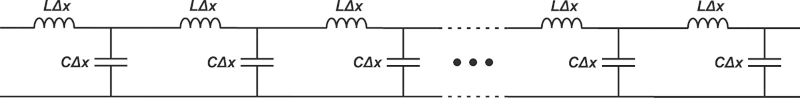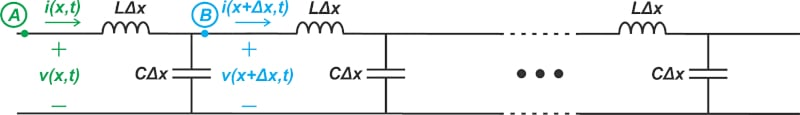Technical Article

# Investigating Lossless Transmission Line Phase Constant and Infinite Bandwidth

January 27, 2023 by Dr. Steve Arar

## Learn how to derive the wave equations for a lossless transmission line and look at its infinite bandwidth and phase constant.

One of the irritating things that can be encountered when you start to learn about RF design is the transmission line effect. Wires that were simply some interconnects in the good old days when you were working on a low-frequency board are now complicated components described by some intimidating equations! This might seem like a nuisance at first; however, as you learn more about transmission lines, you might eventually see them as a blessing in disguise and come to appreciate all those complications. Due to their “distributed” nature, lossless transmission lines can provide infinite bandwidth. This is in stark contrast with our intuition from low-frequency circuits.

In this article, we’ll derive the wave equations for a lossless transmission line and take a look at its remarkable feature of infinite bandwidth. However, before that, let’s explain what we mean by the “distributed” nature of transmission lines.

### Lumped vs. Distributed Regimes

When the circuit dimensions are comparable with the shortest wavelength in the circuit, wires should be treated as transmission lines. This highlights a boundary between two operation regimes for circuit components and interconnects, namely “lumped” and distributed regimes. In the lumped regime, we deal with lower frequencies, and electrical signals are assumed to travel through the wires infinitely fast. The term lumped refers to the fact that we can identify separate capacitors and inductors in some specific regions of the circuit. As an example, consider the passive bandpass filter shown in Figure 1.##### Figure 1. Example diagram of a passive bandpass filter.

In “Region A” in the above diagram, the magnetic energy storage dominates, and thus, this portion of the circuit behaves inductively. On the other hand, in “Region B,” the electrical energy is stored in an electric field, which means that this portion is modeled as a capacitor. In this example, some lumped capacitors, inductors, etc., can be used to model the circuit behavior. The circuit theory and analysis that we are taught in the first semesters of college are actually lumped-element circuit analysis. With lumped circuits, Kirchhoff’s voltage law (KVL) and Kirchhoff's Current Law (KCL) can be easily applied to find the voltage and currents in the circuit.

In contrast, in a distributed regime, we cannot identify separate capacitors and inductors. For example, a uniform lossless wire in the distributed regime is modeled as an infinite ladder network of LC sections (Figure 2).##### Figure 2. Infinite ladder network of LC sections.

This model suggests that each infinitesimally short length of the wire stores energy in the form of both magnetic and electric fields. And energy storage in these two forms is distributed throughout the wire. In this case, we cannot separate the capacitive and inductive parts of the circuit; they are intermingled.

Besides, in the distributed regime, the electrical signals travel as a wave along the wire, meaning that the voltages and currents are a function of time and position along the wire. As a result, we can say that KVL and KCL don’t hold at high frequencies.

In the next section, I’ll try to derive the phase constant equation in a relatively approachable way. If you’re not interested in learning about derivation, you can skip this next section and continue from the “Summary of Equations” section.

### Deriving the Phase Constant Equation

A lossless transmission line can be characterized by two important parameters: the characteristic impedance Z0 and the phase constant β. The characteristic impedance specifies the ratio of the voltage wave to the current wave for an infinitely long line. The phase constant characterizes how the wave changes with position. For a lossless line, Z0 is given by Equation 1:

$Z_0 = \sqrt{\frac{L}{C}}$

##### Equation 1.

To derive an equation for β, we need to find the steady-state voltage and current signals that appear in the ladder network model in Figure 3.##### Figure 3. Ladder network model.

With the voltage and current parameters shown for the first LC section, Kirchhoff’s voltage law produces:

$v(x+ \Delta x, t)-v(x, t)= - L \Delta x \times \frac{d i(x, t)}{dt}$

Dividing both sides by Δx, we have:

$\frac{v(x+ \Delta x, t)-v(x, t)}{\Delta x}= - L \times \frac{d i(x, t)}{dt}$

If we consider the limit of this equation as Δx approaches zero, the expression on the left-hand side actually becomes the derivative of v(x, t) with respect to x. Therefore, the above equation can be rewritten as Equation 2:

$\frac{dv(x, t)}{d x}=- L \times \frac{d i(x, t)}{dt}$

##### Equation 2.

For an infinite line, the ratio of voltage to current at any point along the line is equal to Z0. From Equation 1, we get:

$i(x, t)=\frac{v(x,t)}{Z_0}=v(x,t)\sqrt{\frac{C}{L}}$

By substituting for i(x, t) in Equation 2 yields Equation 3:

$\frac{dv(x, t)}{d x}=- \sqrt{LC} \times \frac{d v(x, t)}{dt}$

##### Equation 3.

Now both sides are in terms of the line voltage, but the left-hand side is the derivative of v(x, t) with respect to position, while the right-hand side includes the derivative of the function with respect to time. Since we want the steady-state response to a sinusoidal excitation (such as vs(t) = Acos(ωt) ), we can use the phasor concept from circuit theory.

For this analysis, we can assume that the input is the complex exponential voltage Aejωt rather than vs(t) = Acos(ωt), we then find the voltage or current signal of interest. Finally, we take the real part of the obtained signal to find the output that would be produced by the actual input vs(t) = Acos(ωt).

When we apply Aejωt to the circuit, the term ejωt appears in all voltage and current quantities. For example, v(x, t) can be considered as V(x)ejωt, where V(x) is a complex value referred to as the phasor of v(x, t). In basic circuit theory, the phasors clearly don’t have a dependency on the position because we are dealing with lumped circuits. However, in the transmission line analysis, we expect the phasor to be a function of position. Substituting v(x, t) with V(x)ejωt, Equation 3 produces:

$\frac{d}{d x}\Big (V(x)e^{j \omega t} \Big )=- \sqrt{LC} \times \frac{d}{dt}\Big (V(x)e^{j \omega t} \Big )$

V(x) is not a function of time, and ejωt is not a function of x. Therefore, using a little algebra, the above equation simplifies to:

$\frac{dV(x)}{d x} =- j \omega \sqrt{LC} \times V(x)$

The solution to this first-order differential equation should be familiar to EEs:

$V(x) =V_0 e^{- j \omega \sqrt{LC}x}$

Where V0 is a constant that can be found from boundary conditions at the input and output ports of the line. From phasor analysis, we know that the real part of V(x)ejωt is the output that we would get if we apply vs(t) = Acos(ωt) to the input. Therefore, our final voltage wave is:

$v(x,t)=Real \text{ } Part \text{ }of \Big (V(x)e^{j \omega t} \Big ) = V_0 cos(\omega t-\omega \sqrt{LC}x)$

Defining the phase constant $$\beta = \omega \sqrt{LC}$$, we obtain:

$v(x,t)= V_0 cos(\omega t-\beta x)$

##### Equation 4.

This is the same wave function we used in the previous article to discuss how a voltage wave travels along a transmission line. Dividing Equation 4 by Z0 gives us the forward current wave as Equation 5:

$i(x,t)= \frac{V_0}{Z_0} cos(\omega t-\beta x)$

### Lossless Transition Line Equation Summary

In the previous section, we derived the equations for forward voltage and current waves. In general, both the forward and reflected waves can be present on the line simultaneously. For a lossless line, the overall voltage and current waves take on the form:

$v(x,t)= A cos(\omega t-\beta x) + B cos(\omega t+\beta x)$

$i(x,t)=\frac{A}{Z_0} cos(\omega t-\beta x)- \frac{B}{Z_0} cos(\omega t+\beta x)$

Where the characteristic impedance Z0 and phase constant β are:

$Z_0 = \sqrt{\frac{L}{C}}$

$\beta = \omega \sqrt{LC}$

### Distributed Effect of Transmission Lines: Desired or a Nuisance?

Due to the wave behavior of high-frequency electrical signals, RF designers are obsessed with matching the load impedance to the characteristic impedance of the line. Without impedance matching, maximum power cannot be transferred to the load, and the large peak voltages created by the standing waves can damage circuit components or interconnects. However, the distributed behavior of transmission lines leads to a remarkably interesting property.

Based on the above analysis, if we excite the line by the sinusoidal vs(t) = Acos(ω1t) that is at an arbitrary frequency ω1, the forward voltage wave would be:

$v(x,t)= V_0 cos(\omega_1 t-\beta x)$

Substituting for β, we get:

$v(x,t)= V_0 cos(\omega_1 t- \omega_1 \sqrt{LC} x)=V_0 cos\Big(\omega_1 ( t- \sqrt{LC} x) \Big)$

The signal that we obtain at a given position x is the same as the input except that it is delayed by $$x \sqrt{LC}$$. This result is valid for any frequency. The only assumption is that the line is lossless and acts as a transmission line. If the delay were frequency dependent, different frequency components of the input would experience different amounts of delay, leading to distortion at the output. For example, if we apply a pulse to a system that has a frequency-dependent delay, the output might be totally distorted because different frequency components reach the output with unequal time shifts.

As the equations show, a lossless transmission line passes all frequency components with the same delay. In other words, the line has infinite bandwidth. If we increase L and C, the delay will increase, but we’ll still have a constant delay for all frequency components. You’ll find this feature even more astonishing if you compare it with our intuition from lumped low-frequency circuits, where increasing capacitor values normally reduces the system bandwidth.

In addition to uniform delay, we should also have a frequency-independent attenuation to have a system with infinite bandwidth. In the above discussion, we assumed that the line is lossless and, therefore, the attenuation is zero for all frequencies.

### What About a Lossy Transmission Line?

In a real-world transmission line, the loss can be contributed to many factors, such as conductor losses (skin effect), dielectric losses, and hysteresis effect. These losses are also frequency-dependent.  However, even with a lossy transmission line, it is possible to adjust the line parameters to have a uniform attenuation and group delay (at least in principle). To learn more about this, you can refer to the book “The Design of CMOS Radio-Frequency Integrated Circuits” by Thomas H. Lee.

### Higher-order Mode Propagation

In addition to loss components, there is another factor that limits the usable bandwidth of a transmission line. As we go to higher and higher frequencies, the wavelength of the signal eventually becomes comparable with the cross-sectional size of the transmission line. In this case, electromagnetic field configurations other than those we normally expect are created. These modes are known as higher-order modes of propagation. The propagation velocity of the higher-order modes differs from that of the dominant (or principal) mode. Therefore, we normally attempt to operate the transmission line below its first higher-order mode. For example, depending on the size of the conductors and the type of dielectric employed, a coaxial line might be specified to operate up to about 18 GHz to avoid propagation of the higher-order modes.